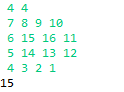# 算法之美-DFS与BFS的比较

DFS与BFS都可以把所有直接或间接相连接的点都遍历到，但是因为机制的不一样所以用处也会有所不同。

DFS(深度优先)一般仅仅用于求一个图有多少个联通分量，看看两个结点之间是否互相连接。

BFS(广度优先)通过队列，把与某个结点直接相连的结点都存入队列中，按照与原结点的距离的近远再依次遍历间接相连的结点。广度优先本身就是用来处理最短与最长路径的问题。

 4 4
7 8 9 10
6 15 16 11
5 14 13 12
4 3 2 1

public class DGraph {
static int[][] arr;
static int MaxCount;
static boolean visited[][];
static int Count;
static int N=0;
static int M=0;
public static void main(String[] args) {
Scanner sc = new Scanner(System.in);
N = sc.nextInt();//点的个数
M = sc.nextInt();
arr = new int[N][M];

for(int j =0;j<N;j++) {
for(int j2=0;j2<M;j2++) {
arr[j][j2] = sc.nextInt();
}
}
for(int j=0;j<N;j++) {
for(int j2=0;j2<M;j2++) {
visited = new boolean[N][M];
Count = 0;
DFS(j,j2);
}
}

System.out.println(MaxCount);

}
private static void DFS(int x, int y) {
// TODO Auto-generated method stub
visited[x][y] = true;
MaxCount = MaxCount>Count?MaxCount:Count;
//up
if(x>=1&&(!visited[x-1][y])&&arr[x-1][y]<arr[x][y]) {
Count++;
DFS(x-1, y);
}
//down
if(x<N-1&&(!visited[x+1][y])&&arr[x+1][y]<arr[x][y]) {
Count++;
DFS(x+1, y);
}
//left
if(y>=1&&(!visited[x][y-1])&&arr[x][y-1]<arr[x][y]) {
Count++;
DFS(x, y-1);
}
//right
if(y<M-1&&(!visited[x][y+1])&&arr[x][y+1]<arr[x][y]) {
Count++;
DFS(x, y+1);
}
visited[x][y] = false;
Count--;
}
}import java.util.ArrayList;
import java.util.List;
import java.util.Queue;
import java.util.Scanner;

public class Skatting2 {
static int[][] Map;
static boolean[] visited;
static int MaxCount;
static int Count;
public static void main(String[] args) {
Scanner scanner = new Scanner(System.in);
int R = scanner.nextInt();
int C = scanner.nextInt();
Map = new int[R][C];
for(int i=0;i<R;i++) {
for(int j=0;j<C;j++) {
Map[i][j] = scanner.nextInt();
}
}
for(int i=0;i<R;i++) {
for(int j=0;j<C;j++) {
if(i-1>=0&&Map[i][j]-Map[i-1][j]>0) {
}
if(i+1<R&&Map[i][j]-Map[i+1][j]>0) {
}
if(j-1>=0&&Map[i][j]-Map[i][j-1]>0) {
}
if(j+1<C&&Map[i][j]-Map[i][j+1]>0) {
}
}
}
MaxCount=0;
for(int i=0;i<totallist.size();i++) {
Count = 0;
visited = new boolean[R*C];
visited[i] = true;
while(!queue.isEmpty()) {
Integer top = queue.poll();
for(Integer item:totallist.get(top)) {
if(!visited[item]) {
Count++;
visited[item] = true;
}
}
}
MaxCount = MaxCount>Count?MaxCount:Count;
}
System.out.println(MaxCount);

}
}

02-10329
11-1614万+09-0356
07-131万+
08-056238
05-026159
03-213万+
06-06247
01-10798
02-281万+